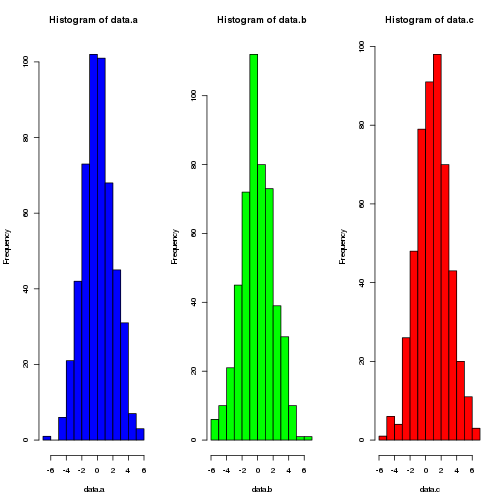## Comparing distributions with nested sampling

A common problem in the analysis of experimental data is deciding whether two sets of data samples represent the same underlying process (for example, when deciding whether a particular treatment has had an effect). One way to do this is by using a t-test. However, we can get use nested sampling to solve this problem instead.

``````library(babar)
``````

### Setting conditions

Then, we'll generate some data. We'll set a number of samples:

``````n.samples <- 500
``````

and set two different means (and a common standard deviation):

``````sd.1 <- 2
mean.1 <- 0

sd.2 <- 2
mean.2 <- 1
``````

### Generating data

Now we'll generate three sets of samples. Two have the same mean and standard deviation and the third is different:

``````data.a <- rnorm(n.samples, mean.1, sd.1)
data.b <- rnorm(n.samples, mean.1, sd.1)
data.c <- rnorm(n.samples, mean.2, sd.2)
``````

### Plotting the data

Let's look at the data:

``````par(mfrow=c(1, 3))
hist(data.a, col='blue')
hist(data.b, col='green')
hist(data.c, col='red')
``````### Comparing the data

Now we can use nested sampling. Here, we use a convenience function that will compare the evidence for the two models:

1. The data samples are drawn from the same normal distribution (same mean and standard deviation).
2. The data are from two different normal distributions (the mean and standard deviations).

The function works by calculating the evidence for each model and returning the difference. Firstly, we can look at the evidence that the first two sets of samples are from the same distribution:

``````compareDistributions(data.a, data.b)
``````
``````##  5.313594
``````

By the Jeffrey's scale, this is substantial evidence in favour. Comparing the first and third:

``````compareDistributions(data.a, data.c)
``````
``````##  -17.56065
``````

We see that the evidence is strongly against the two sets of samples being from the same distribution (the correct result, given that their means differ).

h